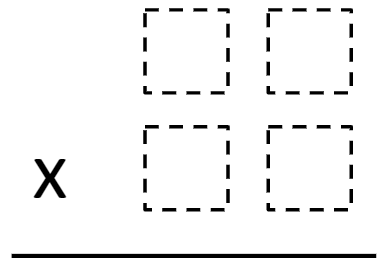# Multiplying Two-Digit Numbers – Closest to 7,000

Directions: Directions: Using the digits 1 to 9 at most one time each, fill in the boxes to make the product as close to 7,000 as possible### Hint

Estimate – what can you multiply to get close to 7,000?

One answer is 96 x 73 = 7,008

Source: Paolo Tolomeo

## Simple Patterns 2

Directions: Using the digits 1 to 9 at most one time each, place a digit …

1.My students discovered another correct response! 92×76 = 6,992

•Anirudh Vebkataraman

I can only find the answer 6,992 too

••2.(3*2)+(4*8)

3.98×71=6958

4.96×74=7,104 that the close i can get.👍

5.Danitza Marenco

I got 92×76=6,992

6.I got 91 times 77= 7007

•You can’t use the 7 twice

•why can’t she?

•The directions said each digit should be used at MOST one time.

7.Courtney Gullickson

My student came up with 92 X 76 = 6992

•Praveen kulkarni

I came up with 96 x 73 = 7008

8.89 x 76 = 6764 that’s only 236 away!

9.Good Luck for the upcomingupdate. Thiss article is truly quite interesting
and effective.
Best regards,
Balle Griffin

10.I got 6912 (72 x 96)

11.use the digits 1 to 9, at most one time each, to make 5 prime numbers.

12.I used 89×76 and got 6,764

13.14.Brianna Harb
90×77= 6930

15.Brianna came out with this answer.

16.i got 76 times 91=6916.

17.97 X 73 =7081

18.96 X 78=7,400

19.91 x 78 = 7016.

20.77×91=7007

21.75
*
93
——
6,975

22.We found 95*74=7030

23.96×73 7008 YEET

24.25.I got 92×76=6,992 as my closet number to 7,000.

26.91×79=7,189 that’s close

27.75×94=7,050

28.29.30.31.